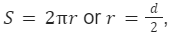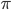• Call Now

1800-102-2727•

# Rules for Significant Figures: Order of Magnitude, Practice Problems, FAQs

A reading of 12.2 cm from a scale whose least count is 1 cm can be trusted? Let’s try to find an answer to this question.

While measuring a physical quantity the reading we take involves some error. So, it should be reported in a way which will describe the precision of the measurement. Usually, the reading is described by a number which includes all the digits which are reliable and the first digit which is uncertain. These all in total are known as the significant digits or significant figures.

Let’s say we are measuring the mass of an object. We measure the mass as 5.43 kg. Here, we are certain up to 5.4 kg and the digit 3 is uncertain.

## Rules For Significant Figures

• All the non-zero digits are significant figures.

1.4514 m has 5 significant figures.

125479 has 6 significant figures.

• All the zeros between two non-0 digits are also significant figures, irrespective of the position of decimal point.

1023404 has 7 significant figures.

2.04007 km has 6 significant figures.

• Zeros left to the extreme left of non-0 digits are not significant figures, irrespective of the position of the decimal point.

0.00412 has 3 significant figures.

008524 g has 4 significant figures.

• The trailing zeros in a number expressed without decimal point are not significant figures.

12300 has 3 significant figures.

4500 has 2 significant figures.

Note: they are just numbers, not any measured quantity and no unit is involved.

• The trailing zeros in a measured quantity expressed without decimal point are significant figures.

12300 kg has 5 significant figures.

4500 m has and 4 significant figures.

• The trailing zeros in a number or a measured quantity with decimal point are significant figures.

1.2300 kg has 5 significant figures.

4.500 m has 4 significant figures.

• The power of 10(i.e. 10x) is not counted as significant figures.

123 and 1.23×102 both have the same i.e., 3 significant figures.

• Changing the units of measurement of a quantity doesn’t change the number of significant figures.

1.200 kg or 1200 g both have the same number of significant figures.

• The multiplying or dividing factor in a measured quantity has an infinite number of significant figures.

For example, inthe constants 2 has an infinite number of significant figures. So this factor 2 can be written in 2.0000 with as many zeros as we wish.

## Order of Magnitude

For convenience, any measurement should be expressed in terms of a×10b where a is in between 1 and 10. b is a positive or negative integer. For example, 2013 km as 2.013×103 km , 25 m as 2.5×10 m, 0.250 kg as 2.5×10-1 kg etc. For approximation a is rounded of to 1 or 10. For, a≤5, it is approximated to 1 and for a>5 it is approximated to 10. Then the form we get is of 10b.The b in 10b is the order of magnitude of the measurement.

## Practice Problems of Rules for Significant Figures

Question.1 In a lab three scientists A, B, C reported the mass of a body as follows:

3.0×102 g, 300 g, 0.3 kg respectively. Comment on their precision.

Answer. The precision can be measured by the number of significant figures in a reading.

In 3.0×102 g, the number of significant figures is 2 as,

a. The power of 10(i.e. 10x) is not counted as significant figures.

b. The trailing zeros in a number or a measured quantity with decimal point are significant figures.

In 300 g, the number of significant figures is 3 as,

c. The trailing zeros in a measured quantity expressed without decimal point are significant figures.

In 0.3 kg, the number of significant figures is 1 as,

d. Zeros left to the extreme left of non-0 digits are not significant figures, irrespective of the position of the decimal point.

The number of significant figures in the readings by A, B, C are 2, 3, 1 respectively.

So, B is more precise in describing the mass of the body.

Question.2 A square shaped chess board is of side length 0.32 m and a football field length is 110 m. Comment and compare on their order of magnitude.

Answer. Chess board has side length of 0.32 m i.e., 3.2×10-1 m approximated to 1×10-1=10-1 m to assess the order of magnitude.

Football field length is 110 m=1.1×102 m approximated to 1×102 m to assess the order of magnitude.

So, football field length is 2-(-1)=3 order of magnitude larger than a chess board.

Question.3  How many significant figures are there in 3462×102 m

a. 2

b. 4

c. 6

d. None of the above

Answer. The power of 10(i.e. 10x) is not counted as significant figures.

So, in the number 3462 there are 4 significant figures.

Question.4  How many significant figures are there in2 ?

Answer.is a constant and it needs an infinite number of significant figures to define it. Same with any arithmetic operation done on it.

## FAQs of Rules for Significant Figures

Question.1 Why are significant figures important when reporting results?

Answer. By using significant figures, we can show how precise a result is. If we express a number beyond the place to which we have actually measured, the reported value is not trustworthy.

Question.2 Are all the zeros between two non-0 digits significant?

Answer. Yes. 2023404 has 7 significant figures.  06007 km has 6 significant figures.

Question.3  The power of 10 (i.e. 10x) is counted as significant figures?

Answer: No, the power of 10 (i.e. 10x) is not counted as significant figures.

Question.4  How many significant figures are there in 34.6209

a. 2

b. 4

c. 6

d. None of the above

Answer. 6. All digits are significant.